Deutsche VersionConversion

Field quantities: e. g. Voltage  "Decibel, Gain, and Loss" ●
gain = amplification and loss = damping
0 dB damping and 0 dB amplification belong to the factor 1

 The reinforcement and also the amplification factor are called simply "gain" and its increase value with amplification is positively designated. Loss or decrease is the damping factor," negative gain" or also "attenuation". Field and energy gain factors, and loss factors are ratios.   Gain is usually defined as the mean ratio of the signal output of a system to the signal input of the same system. It is often expressed using the logarithmic decibel (dB) units ("dB gain"). A gain greater than one is called amplification is the defining property of an active component or circuit, while a passive circuit will have a gain of less than one.

Enter the value for Vout and Vin and press the "=" key.

 Level Lfield = 20 × log10 ( V out ) dB gain -------- --------------- V in "V in" is the reference valueEnergy quantities: e. g. Power "Decibel, Gain, and Loss) ●
Enter the value for P in and P out and press the "=" key.

 Level Lenergy = 10 × log10 ( P out ) dB gain -------- --------------- P in "P in" is the reference value
Conversion: Factor (Ratio) to Gain (Decibels)
and Gain (Decibels) to a Factor (Ratio)

 To use the calculator, simply enter a value. The calculator works in both directions of the ↔ sign.

 ● Factor x (field quantity) ↔ ● Level Lx (voltage, sound pressure)  dB (voltage) gain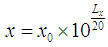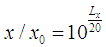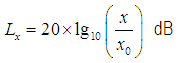Reference factor (field quantity) x0 = 1 ≡ 0 dB (amplitude)

Field quantity x always use the RMS value - but don't do that for the energy quantity y.

 Factor y (energy quantity) ↔ Level Ly (power, sound intensity)  dB (energy) gain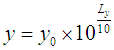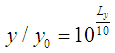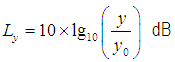Reference factor (energy quantity) y0 = 1 ≡ 0 dB (power)

Energy quantity y is field quantity x (amplitude rms) squared: y ~ x2.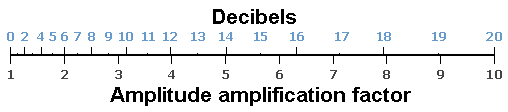Level L in decibel (dB) = 20 × log (x)       x = factor (amplitude, field quantity)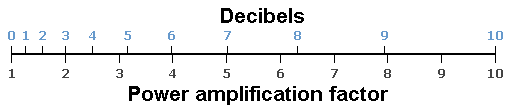Level L in decibel (dB) = 10 × log (y)        y = factor (power, energy quantity)To use the calculator, simply enter a value. The calculator works in both directions of the ↔ sign.

Field quantity (size):

 Voltage V   volt ↔ Voltage level LU  dBV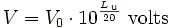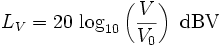Reference voltage V0 = 1 volt (V) ≡ 0 dBV

Energy quantity (size):

 Electric power P   watt ↔ Electric Power level LP  dB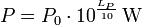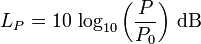Reference power P0 = 1 watt (W) ≡ 0 dB

There is also the reference power P0 = 1 milliwatt or 0.001 watt ≡ 0 dBm

 Electric power (telephone) P   watt ↔ Electric Power level LP  dBmReference power P0 = 1 milliwatt (mW) = 0.001 W ≡ 0 dBm
 Voltage/pressure    Amplification factor 1 1.414  = √2 2 3.16 = √10 4 10 20 40 100 1000 Increasing of x dB 0 3 6 10 12 20 26 32 40 60

 Power/intensity     Amplification factor 1 1.414  = √2 2 3.16 = √10 4 10 20 40 100 1000 Increasing of y dB 0 1.5 3 5 6 10 13 16 20 30The word "power amplifier" is a misnomer. Voltage and current can be amplified. The strange term "power amplifier" has become understood to mean an amplifier that is intended to drive a load such as a loudspeaker. We call the product of current and voltage gain "power amplification".

 Questions: For an amplifier with an amplification factor of 100, calculate the following: a) voltage gain in dB. b) voltage gain at the cutoff frequency (break frequency) in dB. c) amplification factor at the cutoff frequency (break frequency). Answers: a) amplification factor 100 = gain 40 dB. b) gain at the cutoff frequency is 3 dB, so it is 37 dB. c) gain 37 dB = amplification factor 70.7 at the cutoff frequency.